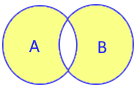# symmetric_difference() method in Python set

All set methods

Returns a new set with all elements and without common elements. Original set remains unchanged.

## symmetric_difference using ^ operatorAll elements except the Common elements in sets
Using caret ( ^ ) operator
``````A={1,2,3}
B={3,4,5}
print(A ^ B)``````
Output ( Note 3 is the only common element so all elements without 3 is returned.)
``{1, 2, 4, 5}``

## Using type()

We can check the output by using type()
``````A={1,2,3}
B={3,4,5}
x=A ^ B
print(type(x))``````
Output
``<class 'set'>``

## Using symmetric_difference() method

``````A={'a','b','c'}
B={'a','y','z'}
print(A.symmetric_difference(B))``````
Output
``{'b', 'y', 'z', 'c'}``

## Using more than one sets

We can use any number of sets with symmetric_difference()
``````A={'a','b','c'}
B={'a','y','z'}
C={'a','k','l'}
print(A ^ B ^ C)``````
Output
``{'y', 'a', 'l', 'b', 'k', 'z', 'c'}``

## symmetric_difference() method

Using string ( iterable object ) with symmetric_difference() method
``````A={'a','b','c','x','y'}
B='Alex'
print(A.symmetric_difference(B))``````
Output
``{'y', 'a', 'l', 'e', 'A', 'b', 'c'}``
Using list with symmetric_difference() method.
``````A={'a','b','c'}
B=['a','x','y']
print(A.symmetric_difference(B))``````
Output
``{'b', 'x', 'c', 'y'}``
Below code will generate error.
``print(A ^ B)``
`` TypeError: unsupported operand type(s) for ^: 'set' and 'list'``

## symmetric_difference_update()

In all above code by using symmetric_difference() we created a new set. By using symmetric_difference_update() method we can change the original set with common elements.

Subscribe to our YouTube Channel here

## Subscribe

* indicates required
Subscribe to plus2netplus2net.com